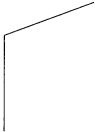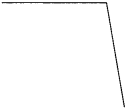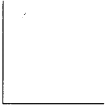Balbharti Maharashtra Board Class 5 Maths Solutions Chapter 6 Angles Problem Set 25 Textbook Exercise Important Questions and Answers.

## Maharashtra State Board Class 5 Maths Solutions Chapter 6 Angles Problem Set 25

Measure the angles given below and write the measure in the given boxes.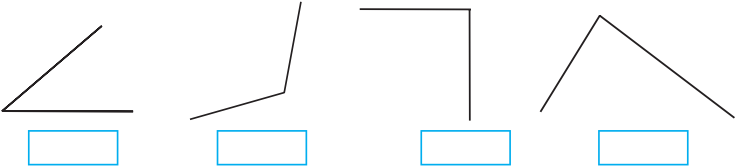(1) 40°
(2) 120°
(3) 90°
(4) 85°Drawing an angle of the given measure
Example Draw ∠ABC of measure 70°.
B is the vertex of∠ABC and BA and BC are its arms.

1. First draw arm BC with a ruler.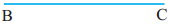2. Since B is the vertex, we must draw a 70° angle at that point.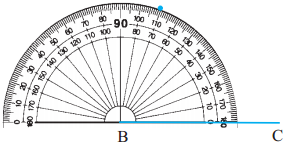Put the centre of the protractor on B. Place the protractor so that the baseline lies on arm BC. Count the divisions starting from the 0 near point C. Mark a point with your pencil at the division that shows 70°. Lift the protractor.

Draw a line from vertex B through the point marking the 70° angle. Name the other end of the line A.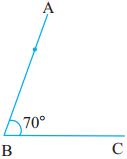∠ABC is an angle of measure 700.
Rahul and Sayali drew ∠PQR of measure 800 as shown below.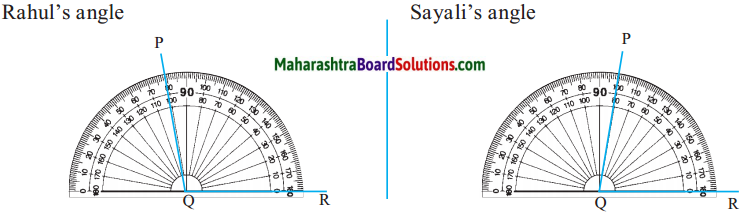Teacher : Have Rahul and Sayali drawn the angles correctly?
Shalaka : Sir, Rahul’s angle is wrong. Sayali’s angle is correct.
Teacher : Why is Rahul’s angle wrong?
Rahul : I counted 10, 20, 30…from the left and drew the angle at 80.
Teacher : Rahul measured the angle from the left. Under the baseline on the left of Q, there is nothing. The arm of the angle is on the right of Q. Therefore, the point should have been marked 80° counting from the right side, that is, on the side on which point R lies.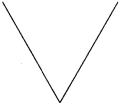60°